# Octal Calculator

To calculate the addition, subtraction, multiplication, and division between two octal numbers. Input the 1st octal and 2nd octal value, select the arithmetic operator between them, and hit the "calculate" button of this octal calculator.

Give Us Feedback

The octal number calculator performs mathematical operations on two decimal numbers according to the octal number system. It allows basic four mathematical operations to be performed. These operations include

1. Sum (+)
2. Difference (-)
3. Multiplication (x)
4. Division (/)

This base 8 calculator converts the input numbers into octal numbers and saves the hassle of the intermediate steps.

## What is the octal system?

There are different number systems in mathematics like binary, octal, decimal e.t.c. All of these systems have their own importance and applications.

The octal system consists of 8 numerical numbers from 0 to 7. There is no 8 or 9 in this similar to the decimal system.

## How to convert into octal numbers?

There are calculators available like decimal to octal and binary to octal. The process is long and requires precision.

Perform the long division by divisor 8 on the number and keep their remainders. Continue dividing until the quotient becomes less than 8 (remainder). Now write the remainders from bottom to top including the last quotient.

## How to perform arithmetic operations on octal numbers?

There is no difficult step involved in performing the operations on octal values, just a little trick. You have to convert the answer into the octal system during the process.

Below, you can find one example for each operation.

Add the numbers 672 and 777.

Solution:

1. Write the addend below the augend and add the first two numbers on the right side.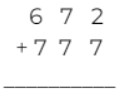2. 7 and 2 add into 9 which is not a digit of the octal system. So, convert it into the octal system by dividing.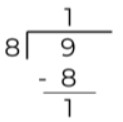3. Write 11 as the sum of 7 and 2 and place 1 in the bottom and the other one as carrying.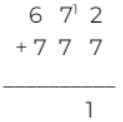4. Similarly, add the remaining two numbers.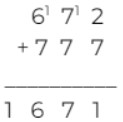So the answer is 1671 in the octal system.

### Octal Subtraction:

It is similar to octal addition. The difference comes in carrying. In the decimal system, when taking a carry from the left side, you add 10 to the right digit. But in the octal system, you have to add 8.

Subtract 434 from 707.

Solution:

1. Write the subtrahend under the minuend and perform subtraction on the first two digits.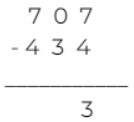2. Take 8 carry from the 7 on the leftmost and add into 0.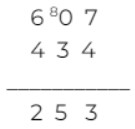Hence, the answer is 253. For octal addition and subtraction, use the base 8 calculator above.

### Octal Multiplication:

One key rule of octal division is that only multiples up to 7 are allowed. Multiply the value 77 with 5.

Solution:

1. Multiply the first 7 with 5. As the answer is 35, well within the octal system, therefore no conversions are required.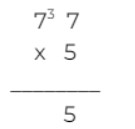2. On second multiplication, the previous 3 is also added. The answer becomes 38. This number on conversion becomes 46.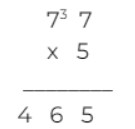### Octal division:

Lastly, an example of octal division. This operation is a bit time-taking. Divide 752 by 4

Solution:

1. The first left digit is 7, a number greater than 4. So, divide.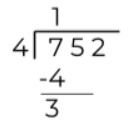2. Now bring the 5 in the bottom and find the suitable multiple of 4 which can divide 35.

4 into 7 is 28 in the decimal system hence it cannot be used. But if it is converted into the octal system, it becomes 34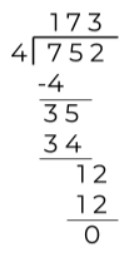Lastly, divide the 12 in the end. The quotient 173.

### Math Tools